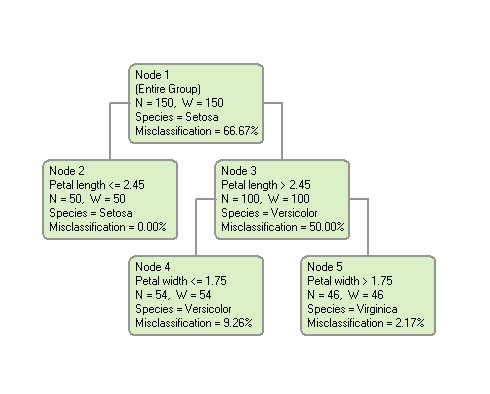### Using a Generated Model to Predict Target Variable Values

One a predictive model has been built with DTREG, it can be used to predict the values of the target variable based on values of the predictor variables.As an example of the score process, we will consider a single decision tree. The Score operation can be used with any type of model. To determine the predicted value of a row, begin with the root node (node 1 above). Then decide whether to go into the left or right child node based on the value of the splitting variable. Continue this process using the splitting variable for successive child nodes until you reach a terminal, leaf node. The value of the target variable shown in the leaf node is the predicted value of the target variable.

For example, let’s use the tree shown above to classify a case that has the following predictor values:

Petal length = 3.5
Petal width = 2.1

Begin the analysis by starting in the root node, node 1. The first split is made using the Petal length predictor. Since the value of Petal length in our case is 3.5, which is greater than the split point of 2.45, we move from node 1 into node 3. If we stopped at that point, the best estimate of Species would be Versicolor. Node 3 is split on a different predictor variable, Petal width. Our value of Petal width is 2.1, which is greater than the split point of 1.75, so we move into node 5. This is a terminal node, so we classify the species as Virginica, which is the category assigned to the terminal node.

In the case of regression trees where the target variable is continuous, the mean value of the target variable for the rows falling in a leaf node is used as the predicted value of the target variable.